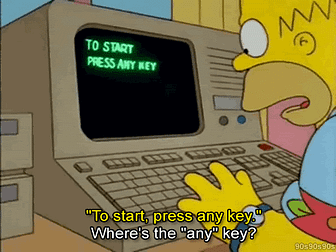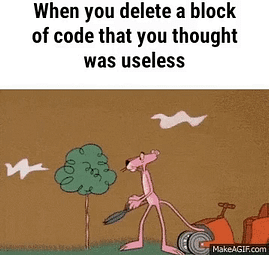Lessons Learned: HashMaps
Question: Invert a binary tree. Input: 4 / \ 2 7 / \ / \ 1 3 6 9 Output: 4 / \ 7 2 / \ / \ 9 6 3 1 Solution Approach 1: Recursive The inverse of an…
Alice and Bob take turns playing a game, with Alice starting first. Initially, there is a number N on the chalkboard. On each player's turn, that player…

### March 2021

Question: Given a non-empty array of integers nums, every element appears twice except for one. Find that single one. Solution Approach 1: Hash Table We…Given an array A of integers, for each integer A[i] we may choose any x with -K <= x <= K, and add x to A[i]. After this process, we have some array B…Lessons Learned: TreeSet, Array Manipulation

### February 2021Question: Implement an algorithm to print all valid (properly opened and closed) combinations of n pairs of parentheses.Lessons Learned: Array parsingThe problem: Given two words (start and end), and a dictionary, find the length of shortest transformation sequence from start to end.
Lessons learned: DFS

### January 2021Question: Given a string s and a dictionary of words dict, determine if s can be segmented into a space-separated sequence of one or more dictionary…
👋 Hello! I’m gathering feedback on SWE Prep. If you like to provide suggestions or feedback, let us know on this survey here! Question: Given a string…